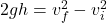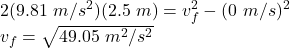Question

what is the velocity of a ball right before it hits the ground if it falls from a 2.5 m bookshelf?

1.thachthao

vf = 7 m/s

Explanation:

The final velocity of the ball right before it hits the ground can be found by using the third equation of motion:where,

g = acceleration due to gravity = 9.81 m/s²

h = height = 2.5 m

vf = final velocity = ?

vi = initial velocity =  0 m/s

Therefore,vf = 7 m/s